Ex 6.5

Chapter 6 Class 10 Triangles
Serial order wise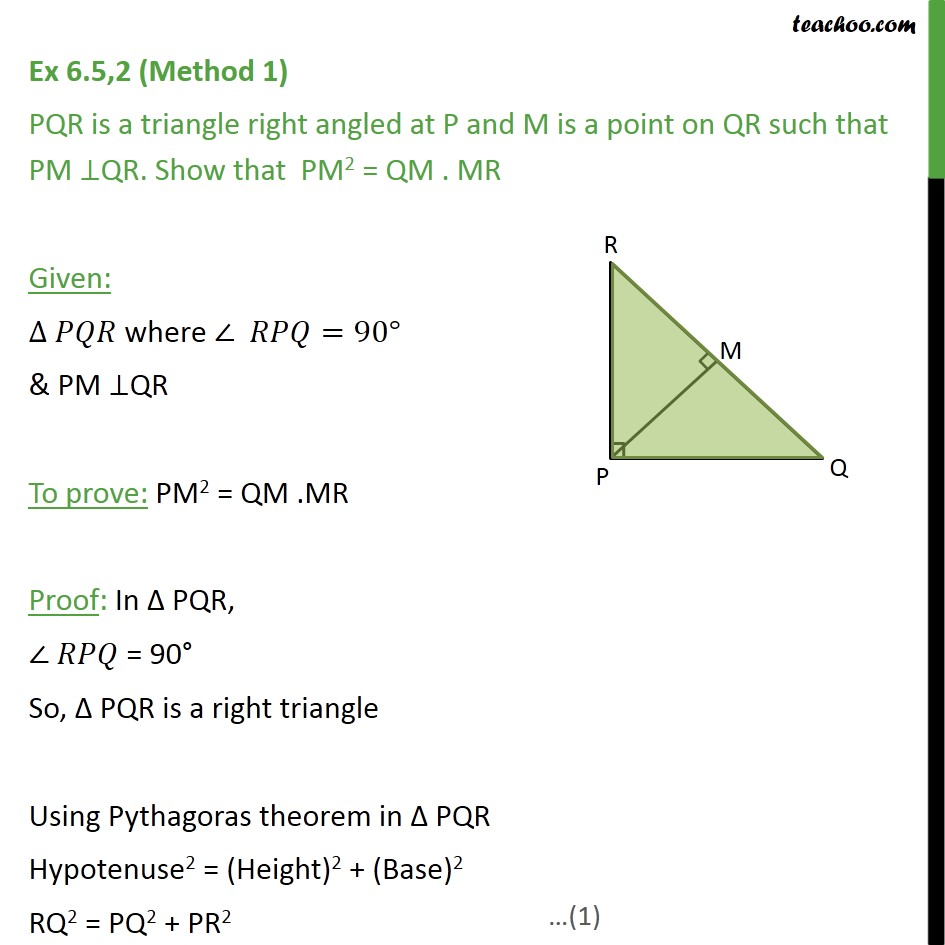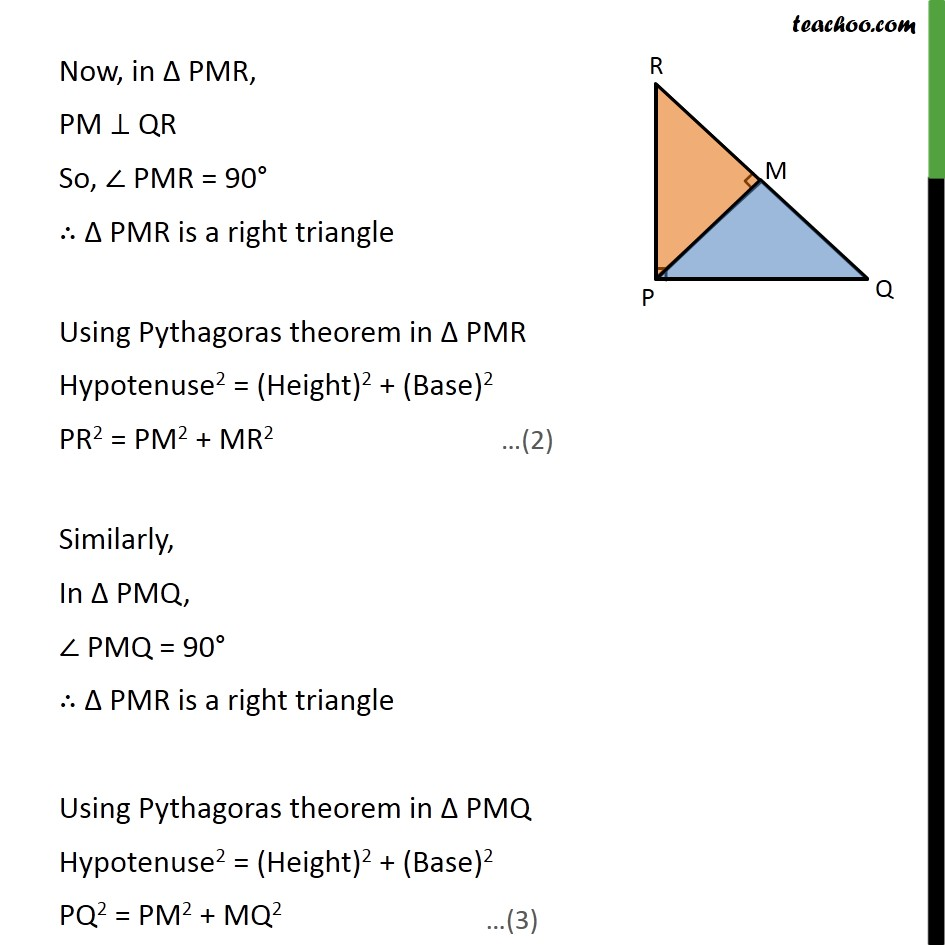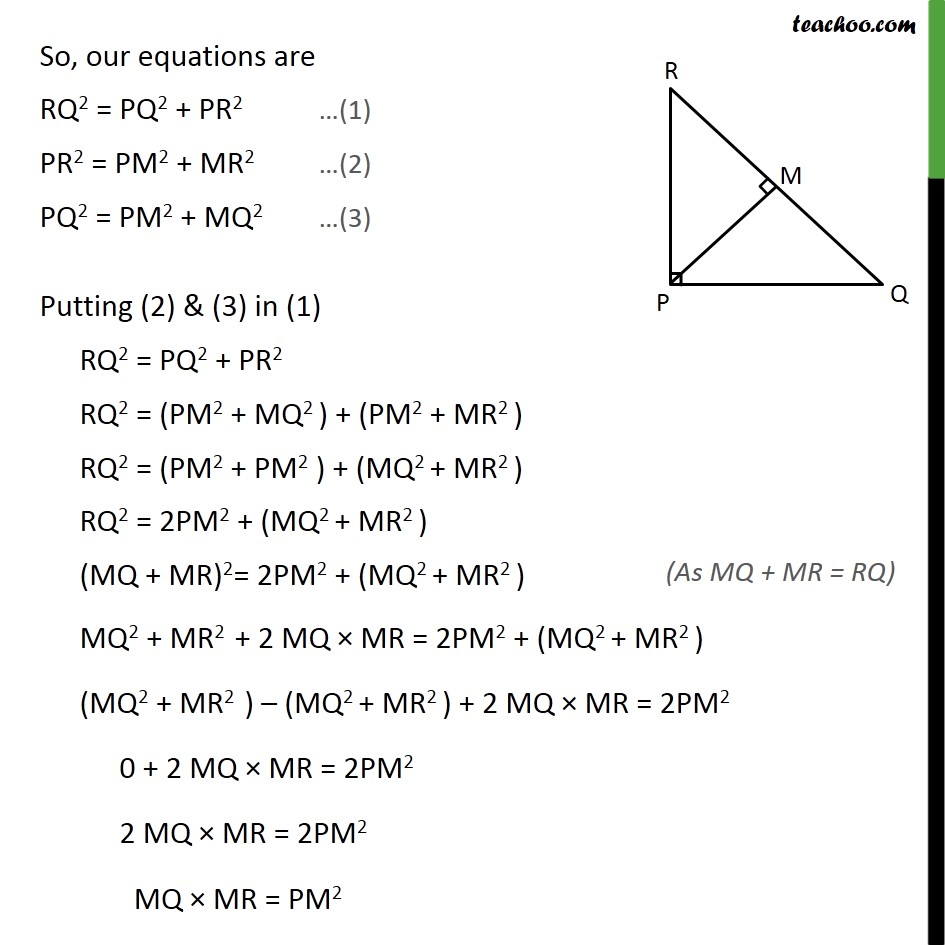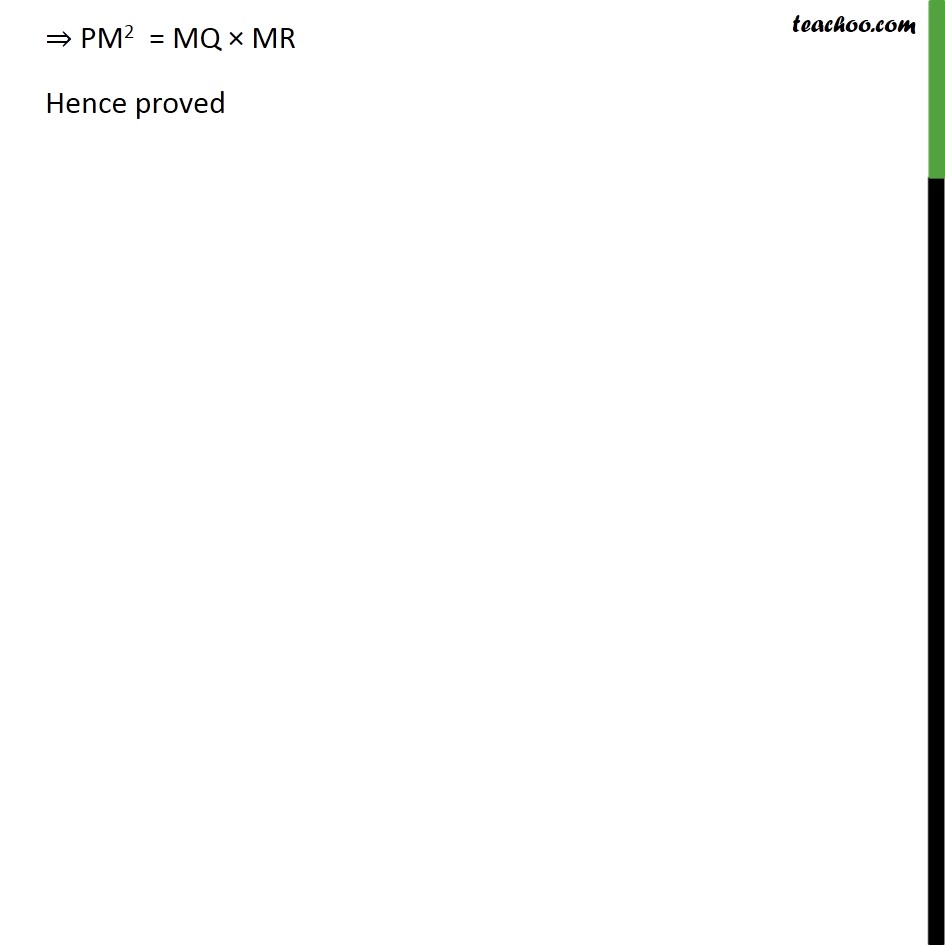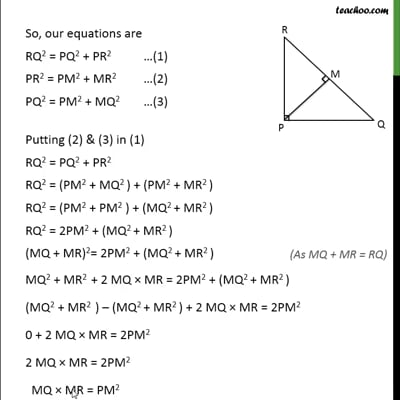This video is only available for Teachoo black users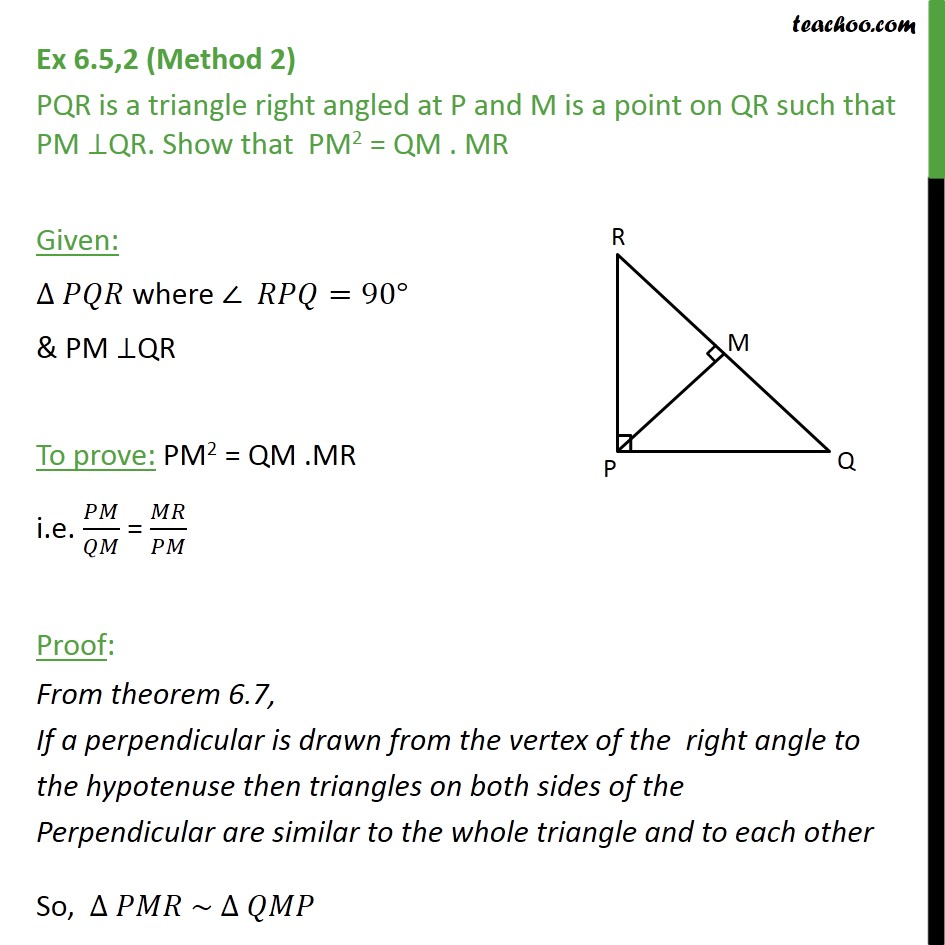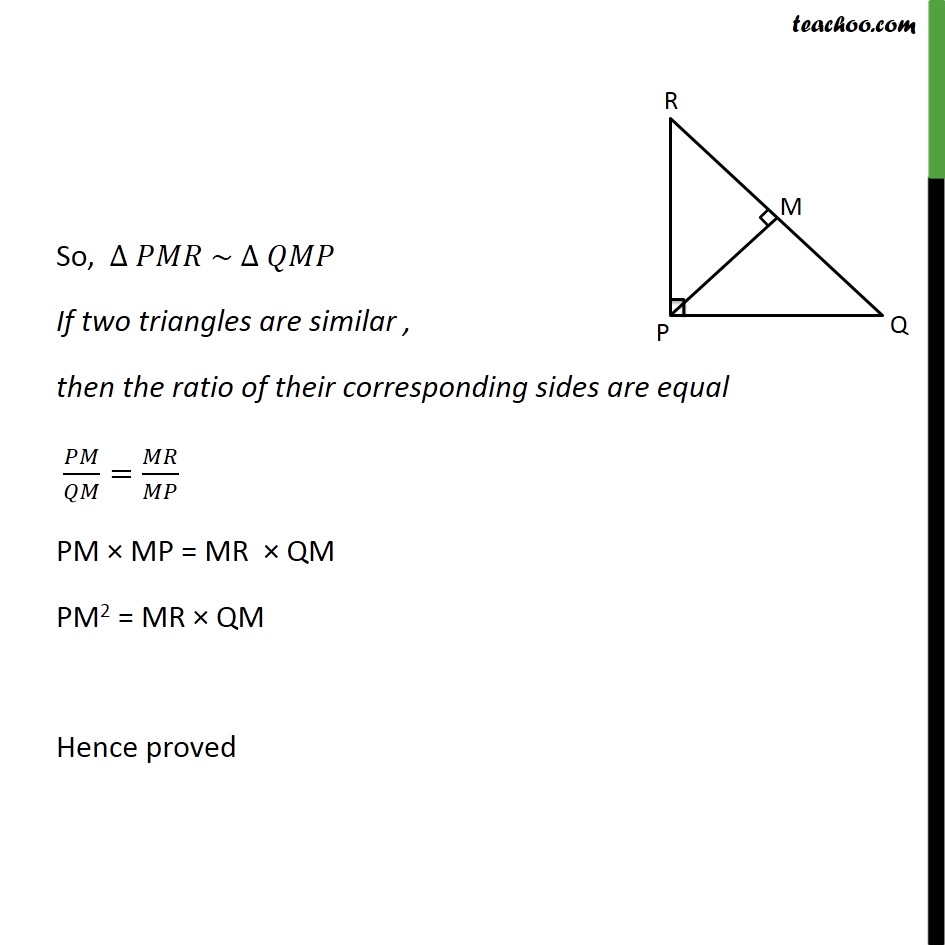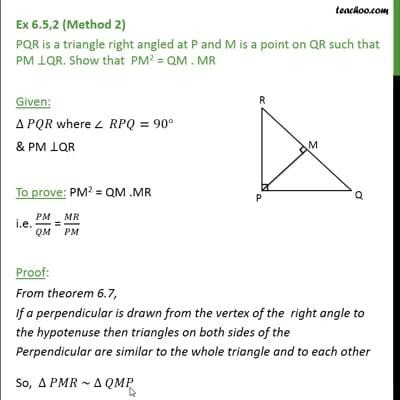This video is only available for Teachoo black users

Solve all your doubts with Teachoo Black (new monthly pack available now!)

### Transcript

Ex 6.5,2 (Method 1) PQR is a triangle right angled at P and M is a point on QR such that PM ⊥QR. Show that PM2 = QM . MR Given: ∆ 𝑃𝑄𝑅 where ∠ 𝑅𝑃𝑄=90° & PM ⊥QR To prove: PM2 = QM .MR Proof: In Δ PQR, ∠ 𝑅𝑃𝑄 = 90° So, Δ PQR is a right triangle Using Pythagoras theorem in Δ PQR Hypotenuse2 = (Height)2 + (Base)2 RQ2 = PQ2 + PR2 Now, in Δ PMR, PM ⊥ QR So, ∠ PMR = 90° ∴ Δ PMR is a right triangle Using Pythagoras theorem in Δ PMR Hypotenuse2 = (Height)2 + (Base)2 PR2 = PM2 + MR2 Similarly, In Δ PMQ, ∠ PMQ = 90° ∴ Δ PMR is a right triangle Using Pythagoras theorem in Δ PMQ Hypotenuse2 = (Height)2 + (Base)2 PQ2 = PM2 + MQ2 So, our equations are RQ2 = PQ2 + PR2 …(1) PR2 = PM2 + MR2 …(2) PQ2 = PM2 + MQ2 …(3) Putting (2) & (3) in (1) RQ2 = PQ2 + PR2 RQ2 = (PM2 + MQ2 ) + (PM2 + MR2 ) RQ2 = (PM2 + PM2 ) + (MQ2 + MR2 ) RQ2 = 2PM2 + (MQ2 + MR2 ) (MQ + MR)2= 2PM2 + (MQ2 + MR2 ) MQ2 + MR2 + 2 MQ × MR = 2PM2 + (MQ2 + MR2 ) (MQ2 + MR2 ) – (MQ2 + MR2 ) + 2 MQ × MR = 2PM2 0 + 2 MQ × MR = 2PM2 2 MQ × MR = 2PM2 MQ × MR = PM2 ⇒ PM2 = MQ × MR Hence proved Ex 6.5,2 (Method 2) PQR is a triangle right angled at P and M is a point on QR such that PM ⊥QR. Show that PM2 = QM . MR Given: ∆ 𝑃𝑄𝑅 where ∠ 𝑅𝑃𝑄=90° & PM ⊥QR To prove: PM2 = QM .MR i.e. 𝑃𝑀/𝑄𝑀 = 𝑀𝑅/𝑃𝑀 Proof: From theorem 6.7, If a perpendicular is drawn from the vertex of the right angle to the hypotenuse then triangles on both sides of the Perpendicular are similar to the whole triangle and to each other So, ∆ 𝑃𝑀𝑅 ~ ∆ 𝑄𝑀𝑃 So, ∆ 𝑃𝑀𝑅 ~ ∆ 𝑄𝑀𝑃 If two triangles are similar , then the ratio of their corresponding sides are equal 𝑃𝑀/𝑄𝑀=𝑀𝑅/𝑀𝑃 PM × MP = MR × QM PM2 = MR × QM Hence proved